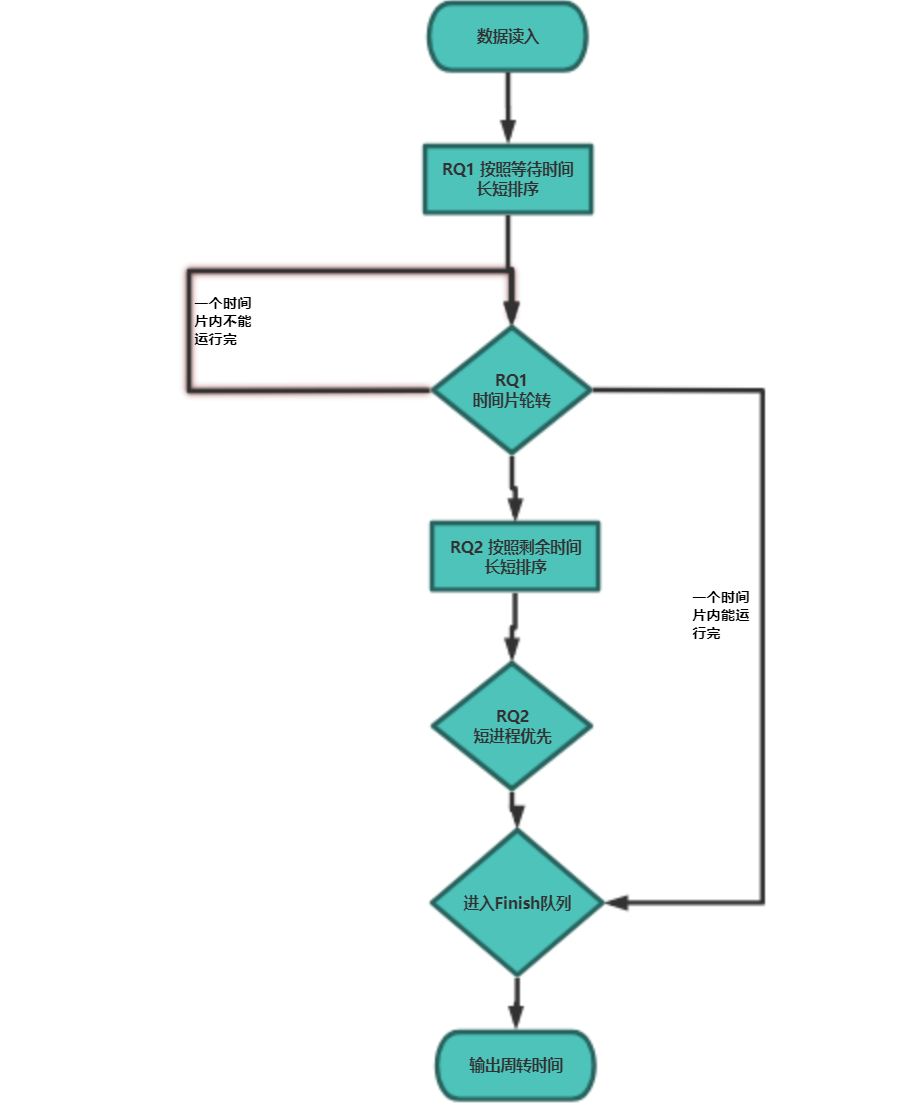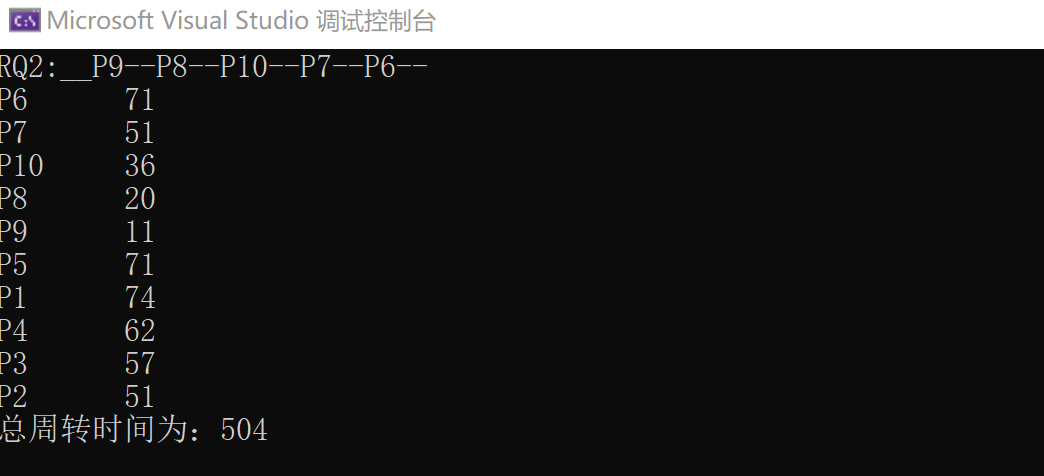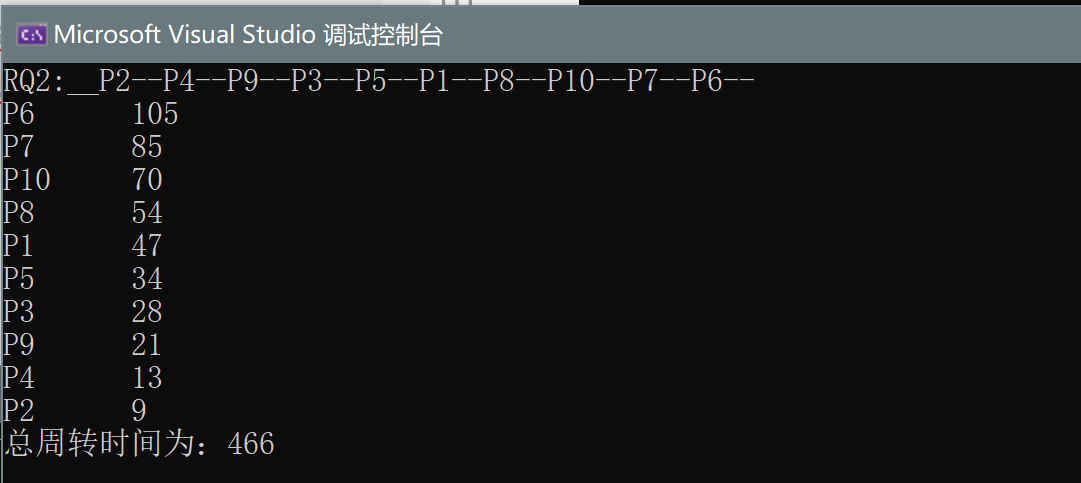• 多级反馈队列调度算法的原理
2021-05-29 18:55:15

多级反馈队列调度算法是一种CPU处理机调度算法，UNIX操作系统采取的便是这种调度算法。

更多相关内容
• C语言实现多级反馈队列调度算法-计算机操作系统实验。C语言实现多级反馈队列调度算法-计算机操作系统实验。
• 多级反馈队列调度算法可以如下原理： 1、设有N个队列（Q1,Q2…QN），其中各个队列对于处理机的优先级是不一样的，也就是说位于各个队列中的作业(进程)的优先级也是不一样的。一般来说，优先级Priority(Q1) > ...

### 多级队列调度算法

• 多级队列：该算法将系统中的进程就绪队列从一个拆分为若干个，将不同类型或性质的进程固定分配在不同的就绪队列，不同的就绪队列采用不同的调度算法，一个就绪队列中的进程可以设置不同的优先级，不同的就绪队列本身也可以设置不同的优先级。
多级队列调度算法由于设置多个就绪队列，因此对每个就绪队列就可以实施不同的调度算法，因此，系统针对不同用户进程的需求，很容易提供多种调度策略。

### 题目描述：

• 设RQ分为RQ1和RQ2
• RQ1采用轮转法，时间片q=7.
• RQ1>RQ2
• RQ2采用短进程优先调度算法。
• 测试数据如下：
• 其中：RQ1: P1-P5,   RQ2: P6-P10

进程P1P2P3P4P5P6P7P8P9P10
运行时间1611141315211810714
已等待时间6543212345

### 程序功能

• 对于给定的数据使用多级队列调度算法进行分析计算周转时间。其中多级队列分为RQ1和RQ2 ，RQ1采用的是时间片长度为7的时间片轮转算法，RQ2采用的是短进程优先算法。并且RQ1的优先级高于RQ2（即只有在RQ1内所有程序运行结束，RQ2才能开始运行）

### 设计思路

• 时间片轮转：首先对RQ1按照等待时间长短排序，然后从头设置循环，只要队列不空就一直进行下去，每次取队头RQ1的下一个元素（RQ1仅用作标志，不存储数据）判断need是否小于等于时间片大小，小于等于则置为0后踢出队列进入finish队列，大于则将need减去时间片大小，然后将其移动至队尾。
• 短进程优先：开始前需要对RQ2按照剩余执行时间大小进行排序，与时间片轮转法类似，不同的是这里一旦开始执行就直接执行完毕，然后下一个进程上处理机运行。### 数据结构

• 本程序每个进程用一个PCB表示，每个PCB内含有name（标识符）、need（当前仍然需要多长时间才能运行结束）、turn（周转时间（等于等待时间+运行时间））、next指针（指向等待队列的下一个进程）。两个队列的头节点分别为RQ1、RQ2还有一个结束队列Finish（运行结束后进程从原队列进入这里）
typedef struct tag_pcb {
char name;
int need = 0;//需要运行的时间
int turn = 0;//周转时间=等待时间+运行时间
struct tag_pcb* next = NULL;
}PCB;
PCB* RQ1=new PCB, * RQ2 = new PCB, * Finish = new PCB;


### 代码实现：#include<iostream>
#include <fstream>
using namespace std;

typedef struct tag_pcb {
char name;
int need = 0;//需要运行的时间
int turn = 0;//周转时间=等待时间+运行时间
struct tag_pcb* next = NULL;
}PCB;
PCB* RQ1=new PCB, * RQ2 = new PCB, * Finish = new PCB;
const int TimePiece = 7;//时间片长度

ifstream In("RQ1.txt");
PCB* Current = RQ1;

while (!In.eof()) {
PCB* Cur = new PCB;

In >> Cur->name >> Cur->need>> Cur->turn;

Current->next = Cur;
Current = Current->next;
}
In.close();

ifstream In1("RQ2.txt");
PCB* Current1 = RQ2;

while (!In1.eof()) {
PCB* Cur1 = new PCB;

In1 >> Cur1->name >> Cur1->need >> Cur1->turn;
Current1->next = Cur1;
Current1 = Current1->next;
}
In1.close();
}

void Q1_Insert(PCB a) { //时间片轮转算法队列的插入(插入尾部)

PCB* Current = RQ1;
while (Current->next != NULL)
Current = Current->next;
Current->next = new PCB;

*Current->next = a;
//Current->next = &a;

Current->next->next = NULL;

}
void Q2_Insert(PCB b) { //短进程优先调度算法队列的插入

PCB* Current = RQ2;
while (Current->next != NULL)
Current = Current->next;
Current->next = new PCB;

*Current->next = b;
Current->next->next = NULL;

}
void Fin_Insert(PCB c) { //短进程优先调度算法队列的插入

PCB* cc = new PCB;
*cc = c;

cc->next = Finish->next;
Finish->next = cc;
}
void Q2_sort(PCB *T) {

PCB* X = new PCB;//用来保存排序后的链表
PCB* p = new PCB;//用来保存当此最小值的前一位
PCB* Current = T->next;
PCB * PreCurrent = T;
PCB* TailX = X;

while (T->next != NULL) {
int tem = 999999;

Current = T->next;
PreCurrent = T;
while (Current != NULL) {
if (Current->need < tem) {
tem = Current->need;

p = PreCurrent;
//cout << "处理" << p->name << p->need << "\n";
}
Current = Current->next;
PreCurrent = PreCurrent->next;
}

TailX->next = p->next;
TailX = TailX->next;

if (p->next->next != NULL)
p->next = p->next->next;
else
p->next = NULL;
}
*T = *X;
}

int main()
{

int clock = 0; //时钟
while (RQ1->next != NULL) {//表示RQ1还有元素
int t = TimePiece;
PCB* Current = RQ1->next;
int fin = 0;

if (Current->need <= t)
t = Current->need, fin = 1;

clock += t;//表示pi运行t

//输出计算过程
//cout << "\n" << Current->name << "_____" << Current->turn << "__+ ___" << clock << "__= ___" << Current->turn +clock << "\n";

Current->need -= t;

if (fin)
Current->turn += clock, Fin_Insert(*Current);//运行结束
else
Q1_Insert(*Current);//进入队尾等待运行

if (Current->next == NULL)
break;
RQ1->next = Current->next;
}

clock = 0;//时钟要清空一次
Q2_sort(RQ2);//先排序

cout << "RQ2:__";
for (PCB* Current2 = RQ2->next; Current2 != NULL; Current2 = Current2->next)
cout << Current2->name << "--";

while (RQ2->next != NULL) {//表示RQ2还有元素(到这一步默认RQ1已经为空)
PCB* Current3 = RQ2->next;
int t = Current3->need;

clock += t;//表示pi运行t
Current3->need -= t;//实质为清空
Current3->turn += clock;

Fin_Insert(*Current3);

if (Current3->next == NULL)
break;
RQ2->next = Current3->next;
}

int SUM = 0;
for (PCB* Current2 = Finish->next; Current2 != NULL; Current2 = Current2->next) {
cout << "\n" << Current2->name <<"\t"<< Current2->turn ;
SUM += Current2->turn;
}

cout << "\n总周转时间为：" << SUM << "\n";
}


• 多级队列调度测试结果：### 附：

多级反馈队列调度算法如下原理：

• 1、设有N个队列（Q1,Q2…QN），其中各个队列对于处理机的优先级是不一样的，也就是说位于各个队列中的作业(进程)的优先级也是不一样的。一般来说，优先级Priority(Q1) > Priority(Q2) > … > Priority(QN)。怎么讲，位于Q1中的任何一个作业(进程)都要比Q2中的任何一个作业(进程)相对于CPU的优先级要高（也就是说，Q1中的作业一定要比Q2中的作业先被处理机调度），依次类推其它的队列。
• 2、对于优先级最低的队列来说，里面是遵循时间片轮转法。也就是说，位于队列QN中有M个作业，它们的运行时间是通过QN这个队列所设定的时间片来确定的；对于其他队列，遵循的是先来先服务算法，每一进程分配一定的时间片，若时间片运行完时进程未结束，则进入下一优先级队列的末尾。
• 3、各个队列的时间片是一样的吗？
不一样，这就是该算法设计的精妙之处。各个队列的时间片是随着优先级的增加而减少的，也就是说，优先级越高的队列中它的时间片就越短。同时，为了便于那些超大作业的完成，最后一个队列QN(优先级最低的队列)的时间片一般很大(不需要考虑这个问题)。

上述程序在某一进程在一级队列运行一轮后没有运行完毕，若加入二级队列而不是加入原队列的尾部，则可以实现简单的多级反馈队列调度算法
两种算法的不同之处就在于：当一个RQ1中的进程在时间片结束之后是回到当前的队尾还是到RQ2队列之中。
在上述程序中也很容易实现：

		if (fin)
Current->turn += clock, Fin_Insert(*Current);//运行结束
else
Q1_Insert(*Current);//进入队尾等待运行


修改为：

		if (fin)
Fin_Insert(*Current);//运行结束
else
Q2_Insert(*Current);//进入二级队列等待运行
Current->turn += clock,


上述两种代码分别实现了上述两种功能，执行时只需选一种在相应位置即可。

• 多级反馈队列调度测试结果：由分析上述数据容易发现：在该测试数据的情况下多级反馈队列调度算法是要优于多级队列调度的

展开全文链表 c++ 操作系统
• 本文件是对操作系统进程多级反馈队列调度算法的设计与实现，算法以txt的形式输入输出，其中包含设计报告
• 用C语言实现的多级反馈队列调度算法，操作系统课程作业。用VC6.0调试通过。
• 多级(假设为N级)反馈队列调度算法可以如下原理： 1、设有N个队列（Q1,Q2…QN），其中各个队列对于处理机的优先级是不一样的，也就是说位于各个队列中的作业(进程)的优先级也是不一样的。一般来说，优先级Priority(Q1...

多级(假设为N级)反馈队列调度算法可以如下原理：
1、设有N个队列（Q1,Q2…QN），其中各个队列对于处理机的优先级是不一样的，也就是说位于各个队列中的作业(进程)的优先级也是不一样的。一般来说，优先级Priority(Q1) > Priority(Q2) > … > Priority(QN)。怎么讲，位于Q1中的任何一个作业(进程)都要比Q2中的任何一个作业(进程)相对于CPU的优先级要高（也就是说，Q1中的作业一定要比Q2中的作业先被处理机调度），依次类推其它的队列。
2、对于优先级最低的队列来说，里面是遵循时间片轮转法。也就是说，位于队列QN中有M个作业，它们的运行时间是通过QN这个队列所设定的时间片来确定的；对于其他队列，遵循的是先来先服务算法，每一进程分配一定的时间片，若时间片运行完时进程未结束，则进入下一优先级队列的末尾。
3、各个队列的时间片是一样的吗？不一样，这就是该算法设计的精妙之处。各个队列的时间片是随着优先级的增加而减少的，也就是说，优先级越高的队列中它的时间片就越短。同时，为了便于那些超大作业的完成，最后一个队列QN(优先级最低的队列)的时间片一般很大(不需要考虑这个问题)。

展开全文• 短学期课程设计 多级反馈队列调度算法的实现 可供参考
• 包含各种多级反馈队列调度算法，C代码，尤其是抢占！

近期发现，网上对于多级反馈队列调度算法十分匮乏，要么代码太老，要么没什么用，限制性太大，要么copy来copy去，真没意思。正好自己操作系统上课要用，特此分享。
根据需求，自己可以在主函数中选择调度算法，包含抢占算法

	//RR(SLICE);
// FCFS();
//SJF();
//SRTF();
//NonPriority();
Priority();


### 完整代码

#include<stdio.h>
#include<stdlib.h>
#include<stdbool.h>
#include<unistd.h>
#include<time.h>
#include<string.h>
#include <sys/types.h>
// #include<sys/syscall.h>
/*本次实验模拟实现操作系统中进程调度算法,模拟进程在不同时刻到达的情况*/
#define PNUM  5 //进程的数量
#define TIMER 10 //定时器,最长CPU区间时间
#define SLICE 2//轮转算法的时间片
int timenow=0;     //当前时刻
typedef struct node{
int pid;   //进程号
int priority;//进程优先级,1~3,数字越小优先级越高
int arrival; //到达时间
int burst;  //CPU区间时间
int rest;  //剩余时间
char state;//进程状态,'N'新建,'R'运行,'W'等待CPU(就绪),'T'终止
struct node *next;
}PCB;

int gantt[TIMER*PNUM]={0}; //用一个gantt数组记录调度过程,每个时刻调度的进程号

PCB *job;//所有作业的序列,带头节点(为简化编程)
PCB *tail=NULL;  //记录就绪队列的尾节点
PCB *run=NULL;//正在运行中的进程,不带头结点
PCB *finish=NULL;//已经结束的程序,不带头结点

void InitialJob()
{
int i=0;
PCB *p,*tail;
job=(PCB *)malloc(sizeof(PCB));//生成头节点,其它域无意义
job->next=NULL;
tail=job;

for(i=0;i<PNUM;i++)         //初始化进程的值
{ p=(PCB *)malloc(sizeof(PCB));//生成新节点(新进程)
p->pid=i+1;
p->priority=rand()%3+1;//随机生成优先级:1~3
p->arrival=rand()%TIMER;//随机生成到达时刻0-9,(预计到达就绪队列的时间)
p->burst=rand()%TIMER+1;//随机生成CPU区间时间:1~10;(估计运行时间)
p->rest=p->burst;
p->state='N';//初始化进程的状态为'新建'
p->next=NULL;
tail->next=p;
tail=p;  //带头结点
}
}
void DisplayPCB(PCB *pcb) //显示队列
{
struct node *p=pcb;
if(pcb==NULL) {printf("XXXXXX\n");return;}
printf("进程号 优先级 到达时刻 区间时间 剩余时间 进程状态\n");
do{
printf("P%-3d\t",p->pid);
printf("%3d\t",p->priority);
printf("%3d\t",p->arrival);
printf("%3d\t",p->burst);
printf("%3d\t",p->rest);
printf("%3c\t",p->state);
printf("\n");
p=p->next;
}while(p!=NULL);
}

void DisplayGantt() //显示甘特数组
{
int i=0;
for(i=0;i<timenow;i++)
{
if(gantt[i]==0) printf("空闲,");
else
printf("P%d,",gantt[i]);
}
printf("\n");
}

/*注:关于周转时间,等待时间与响应时间的概念释疑:
在课程教材<操作系统概念第7版>中(P141),上述三个时间是从进程到达的时间开始的.
在辅助教材<现代操作系统第4版>中(P89),上述三个时间时从进程提交的时刻(0时刻)开始的.
国内普遍接受前一种理解,本程序以课程教材中的解释为准来计算时间.
*/
void DisplayTime() //显示周转时间t,等待时间w和响应时间r
{
int t=0,w=0,r=0;
float t_avg=0,w_avg=0,r_avg=0;
int i,j;
PCB *p; //用p遍历finish队列,查找进程Pi的到达时间,调用该函数时所有进程都已放入finish队列
if(finish==NULL) {return;}
printf("进程号    周转时间    等待时间    响应时间\n");
for(i=1;i<=PNUM;i++)
{ p=finish;
while(p->pid!=i) p=p->next;
j=0;
while(gantt[j]!=i) j++; //遍历甘特数组,求进程Pi的响应时间
r=j;  //响应时刻
t=j+1;
for(j=r+1;j<timenow;j++) //继续遍历,求周转时间
{ if(i==gantt[j]) t=j+1;}//结束时刻
r=r-p->arrival;  //响应时间=响应时刻-到达时刻
t=t-p->arrival; //周转时间=结束时刻-到达时刻
w=t-p->burst; //等待时间=周转时间-运行时间
r_avg+=(float)r/PNUM; //平均响应时间
w_avg+=(float)w/PNUM;  //平均等待时间
t_avg+=(float)t/PNUM;   //平均周转时间

printf("P%d       %4d       %4d       %4d\n",i,t,w,r);
}
printf("平均周转时间:%.2f,平均等待时间%.2f,平均响应时间%.2f\n",t_avg,w_avg,r_avg);
}
void ReadyQueue(char * algo,int t) //根据作业队列构造就绪队列,algo:算法,t:当前时刻
{
struct node *jpre,*jcur,*rpre,* rcur;
int j,r,a=0;
if(strcmp(algo,"FCFS")==0||strcmp(algo,"RR")==0)//FCFS和RR的就绪队列的构造方式相同
a=0;
else if(strcmp(algo,"SJF")==0)  //非抢占SJF
a=1;
else if(strcmp(algo,"SRTF")==0)  //抢占式SJF,最短剩余时间优先
a=2;
else if(strcmp(algo,"Priority")==0||strcmp(algo,"NonPriority")==0)//抢占和非抢占优先级
a=3;
if(job->next==NULL) {printf("作业队列为空!\n");return;}
jpre=job;
jcur=job->next;
while(jcur!=NULL) //遍历作业序列中选择已到达进程,将其从作业队列移入就绪队列,直到作业队列为空
{
if(jcur->arrival<=t) //如果当前时刻进程已经到达,则将其插入到就绪队列的合适位置
{
printf("P%d到达.\n",jcur->pid);
jpre->next=jcur->next;  //将jcur从作业队列移除
jcur->state='W';//将进程状态设置为就绪
else  //就绪队列不为空,遍历就绪队列,将jcur插入到合适位置
switch (a){ //遍历就绪队列查找插入点
case 0:    //FCFS,RR.根据到达时间arrival查找合适插入点
while((rcur!=NULL)&&(jcur->arrival>=rcur->arrival))
{rpre=rcur;rcur=rcur->next;}
break;
case 1: //SJF,根据区间时间burst查找合适插入点
while((rcur!=NULL)&&(jcur->burst>=rcur->burst))
{rpre=rcur;rcur=rcur->next;}
break;
case 2:  //STRF,根据剩余时间rest查找合适插入点
while((rcur!=NULL)&&(jcur->rest>=rcur->rest))
{rpre=rcur;rcur=rcur->next;}
break;
case 3:  //Priority, Non-Priority,根据优先级查找合适插入点
while((rcur!=NULL)&&(jcur->priority>=rcur->priority))
{rpre=rcur;rcur=rcur->next;}

break;
default: break;
}
if(rcur==NULL)// 插入点在就绪队列尾部
{
jcur->next=NULL;
rpre->next=jcur;
tail=jcur;
}
{
jcur->next=rcur;
}
else //插入到rpre和rcur之间
{
jcur->next=rcur;
rpre->next=jcur;
}
}
jcur=jpre->next;  //下一个作业
}else   //当前作业未达到
{jpre=jcur;jcur=jcur->next;} //下一个作业
}
printf("\n作业队列:\n");
DisplayPCB(job->next);
}
void FCFS( )
{
timenow=0;
while(true){
printf("\n当前时刻:%d\n",timenow);
printf("就绪队列:\n");
{
if(run==NULL)//若CPU空闲
{
run->next=NULL;
printf("\nP%d被调度程序分派CPU!\n",run->pid);
}
run->rest--;    //修改进程PCB
run->state='R';
printf("\nP%d正在运行.......\n",run->pid);
printf("运行进程:\n");
DisplayPCB(run);
gantt[timenow]=run->pid; //记录当前时刻调度进程的ID号
if(run->rest==0)
{   printf("\nP%d结束!\n",run->pid);
run->state='T';
run->next=finish;   //新完成的节点插入到finish的头结点,简单一点
finish=run;
run=NULL;
printf("结束进程队列:\n");
DisplayPCB(finish);
}
}
timenow++; //下一时刻继续扫描作业队列
}
}
void RR(int slice)  //时间片作为参数
{
timenow=0;
int count=0; //时间片计数,count==slice表示进程已经运行一个时间片
while(true){
printf("\n当前时刻:%d\n",timenow);
printf("就绪队列:\n");

if(tail!=NULL) printf("就绪队列尾节点:P%d\n",tail->pid);
{
if(run==NULL)//若CPU空闲
{
count=0;
run->next=NULL;
printf("\nP%d被调度程序分派CPU!\n",run->pid);
}
count++;
run->rest--;    //修改进程PCB
run->state='R';
printf("\nP%d正在运行.......\n",run->pid);
printf("运行进程:\n");
DisplayPCB(run);
gantt[timenow]=run->pid; //记录当前时刻调度进程的ID号
printf("\ncount=%d\n",count);
if(run->rest==0) //进程结束,将进程设置为终止状态,移入结束进程队列
{   printf("P%d结束!\n",run->pid);
// count=0;
run->state='T';
run->next=finish;   //新完成的节点插入到finish的头结点,简单一点
finish=run;
run=NULL;
printf("结束进程队列:\n");
DisplayPCB(finish);
}
else if(count==slice) //时间片结束,进程未结束,将run插入就绪队列尾部
{
printf("P%d时间片结束!\n",run->pid);
// count=0;
run->state='W';
{tail->next=run;
tail=run;
}
else //时间片结束时就绪队列为空,将run直接放入就绪队列
run=NULL;
}
}
timenow++; //下一时刻继续扫描作业队列
}
}
void SJF() //课后完成,其实和FCFS的代码完全相同,除了构造就绪队列时按照短作业排序即可
{
timenow=0;
while(true){
printf("\n当前时刻:%d\n",timenow);
printf("就绪队列:\n");
{
if(run==NULL)//若CPU空闲
{
run->next=NULL;
printf("\nP%d被调度程序分派CPU!\n",run->pid);
}
run->rest--;    //修改进程PCB
run->state='R';
printf("\nP%d正在运行.......\n",run->pid);
printf("运行进程:\n");
DisplayPCB(run);
gantt[timenow]=run->pid; //记录当前时刻调度进程的ID号
if(run->rest==0)
{   printf("\nP%d结束!\n",run->pid);
run->state='T';
run->next=finish;   //新完成的节点插入到finish的头结点,简单一点
finish=run;
run=NULL;
printf("结束进程队列:\n");
DisplayPCB(finish);
}
}
timenow++; //下一时刻继续扫描作业队列
}
}
void SRTF()
{   timenow=0;
PCB *rcur;//用于发生抢占时,遍历就绪队列,将被抢占的进程插入到就绪队列中合适的地方
while(true){
printf("\n当前时刻:%d\n",timenow);
printf("就绪队列:\n");
{
if(run==NULL)//若CPU空闲
{
run->next=NULL;
printf("\nP%d被调度程序分派CPU!\n",run->pid);
}
{   //run被就绪队列的首进程抢占)
while(rcur->next!=NULL&&rcur->next->rest <= run->rest) rcur=rcur->next;
run->state='W';
run->next=rcur->next;  //将run插入到就绪队列中rcur的后面
rcur->next=run;
run->next=NULL;
}
run->rest--;    //修改进程PCB
run->state='R';
printf("\nP%d正在运行.......\n",run->pid);
printf("运行进程:\n");
DisplayPCB(run);
gantt[timenow]=run->pid; //记录当前时刻调度进程的ID号
if(run->rest==0)
{   printf("\nP%d结束!\n",run->pid);
run->state='T';
run->next=finish;   //新完成的节点插入到finish的头结点,简单一点
finish=run;
run=NULL;
printf("结束进程队列:\n");
DisplayPCB(finish);
}
}
timenow++; //下一时刻继续扫描作业队列
}
}
void NonPriority() //非抢占优先级
{
timenow=0;
while(true){
printf("\n当前时刻:%d\n",timenow);
printf("就绪队列:\n");
{
if(run==NULL)//若CPU空闲
{
run->next=NULL;
printf("\nP%d被调度程序分派CPU!\n",run->pid);
}
run->rest--;    //修改进程PCB
run->state='R';
printf("\nP%d正在运行.......\n",run->pid);
printf("运行进程:\n");
DisplayPCB(run);
gantt[timenow]=run->pid; //记录当前时刻调度进程的ID号
if(run->rest==0)
{   printf("\nP%d结束!\n",run->pid);
run->state='T';
run->next=finish;   //新完成的节点插入到finish的头结点,简单一点
finish=run;
run=NULL;
printf("结束进程队列:\n");
DisplayPCB(finish);
}
}
timenow++; //下一时刻继续扫描作业队列
}
}
void Priority()//抢占式优先级
{
timenow=0;
PCB *rcur;//用于发生抢占时,遍历就绪队列,将被抢占的进程插入到就绪队列中合适的地方
while(true){
printf("\n当前时刻:%d\n",timenow);
printf("就绪队列:\n");
{
if(run==NULL)//若CPU空闲
{
run->next=NULL;
printf("\nP%d被调度程序分派CPU!\n",run->pid);
}
{   //run被就绪队列的首进程抢占)
while(rcur->next!=NULL&&rcur->next->priority <= run->priority) rcur=rcur->next;
run->state='W';
run->next=rcur->next;  //将run插入到就绪队列中rcur的后面
rcur->next=run;
run->next=NULL;
}
run->rest--;    //修改进程PCB
run->state='R';
printf("\nP%d正在运行.......\n",run->pid);
printf("运行进程:\n");
DisplayPCB(run);
gantt[timenow]=run->pid; //记录当前时刻调度进程的ID号
if(run->rest==0)
{   printf("\nP%d结束!\n",run->pid);
run->state='T';
run->next=finish;   //新完成的节点插入到finish的头结点,简单一点
finish=run;
run=NULL;
printf("结束进程队列:\n");
DisplayPCB(finish);
}
}
timenow++; //下一时刻继续扫描作业队列
}
}
int main()
{    srand((int)time(NULL)); //随机数种子
//srand(0);
InitialJob();
DisplayPCB(job->next);
//RR(SLICE);
//  FCFS();
//SJF();
//SRTF();
//NonPriority();
Priority();
DisplayGantt();
DisplayTime();
return EXIT_SUCCESS;;
}

展开全文c语言 数据结构
• 操作系统原理多级反馈队列调度算法Multilevel feedback，多处理器调度算法考虑的问题，常见操作系统上的调度算法，Windows中IO线程和“饥饿”线程优先级提升 一、多级反馈队列调度算法Multilevel feedback 1、设置...系统架构
• 多级反馈队列进程调度GUI实现，使用Swing编写的一个可视化界面，支持进程的动态创建，进程调度过程可视化。
• This model paper was revised by LINDA on December 15...多级反馈队列调度算法的实现学生实习报告课程名称_ 数据结构与数据处理应用训练题目名称 多级反馈队列调度算法的实现学生学院 计算机与计算科学专业班级学 ...
• 在Linux下编程实现多级反馈队列调度算法，采用三级调度策略，所有进程先按到达顺序进入一级队列，按照时间片为2轮转一次，一个时间片内未完成的进程被依次移入二队列尾部。当一级队列中没有进程时，开始调度二级队列...
• 多级反馈队列调度算法多级调度算法区别 多级调度算法减去了多级反馈队列算法的调度功能： 在多级调度算法中，系统中会将进程进行优先级分类，比如优先级分类（A,B,C,D） 优先级A>B>C>D，高优先级A的...数据结构
• 请教多级反馈队列调度算法????????????在某一操作系统中对进程调度采用多级反馈队列调度算法。现设定采用三级分数给小编了，小编来 0时刻A到达，进入I队列，执行2个时间段后，转向队列II，再执行了3个时间段后，B...
• 实验一 多级反馈队列调度算法 一. 主要实现方法和代码介绍 ​ 1.编写进程类,其只包含所需的运行时间和进程编号两个属性,还有一个运行方法,此方法就是将所需的运行时间属性减去.传入的运行时间. ​ 2.创建进程函数:...c++
• 时间片轮转调度算法（RR）是十分简单的进程调度算法。 进程在执行时的情况 在该时间片内进程执行完毕，这种情况调度程序将立即把该进程弹出队列，并把CPU分配给新的队首进程 在该时间片内进程未执行完毕，调度程序...java 操作系统
• 1、进程在进入待调度队列等待时，首先进入优先级最高的Q1等待。 2、首先调度优先级高的队列中的进程。若高优先级中队列中已没有调度的进程，则调度次优先级队列中的进程。 3、对于同一个队列中的各个进程，按照...
• 操作系统课程设计报告-多级反馈队列调度算法模拟，操作系统，多级就绪队列，进程调度，时间片轮转法，附带详细的文档说明和源代码
• 进程调度的设计与实现 目录 一、 实验的目的………………………………………………1 二、 实验的内容（任务）及要求……………………………1 三、 实验设备及环境…………………………………………1 四、 实验的原理...
• 下面我们首先介绍，多级反馈队列调度算法 然后对前面介绍的各种调度算法进行比较 之后呢，我们简单讨论一下 在设计多处理器调度算法时所要考虑的几个问题 多级反馈队列调度算法 是 UNIX 的一个分支，BSD 5.3 版所...
• 用c语言模拟实现的多级反馈队列算法，固定进程数，可以自己改写通过键盘输入创建进程数
• 1、设置 N 个就绪进程队列，即队列 0，队列 1，……，队列 N-1，用于存放就绪进程。每个队列 优先级不同，且从队列 0 到...3、在队列内部，进程之间采用先来先服务（FCFS）算法辅以时间片限时机制进行调度：位于队...操作系统...